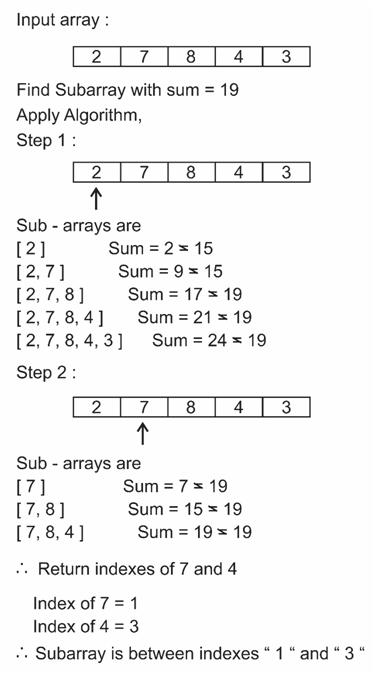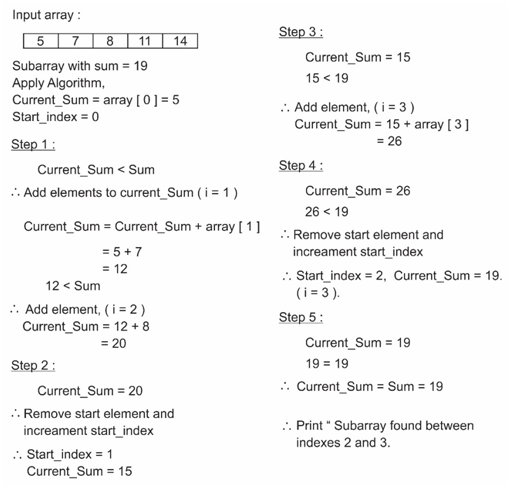# Subarray with given sum

## In the given array with positive integers, find the subarray in which elements add to a given sum.

### Example

a. Input array be: [1, 3, 7, 9, 11, 15, 8, 6]

Sum = 19
Output will be: 1 and 3 → [3, 7, 9], subarray sum = 19

b. Input array be: [1, 3, 7, 9, 11, 15, 8, 6]

Sum = 20
Output will be: 0 and 3 → [1, 3, 7, 9], subarray sum = 20

c. Input array be: [1, 3, 7, 9, 11, 15, 8, 6]

Sum = 40
Output will be: No subarray with given sum found.

d. Input array be: [4, 3, 2, 8, 9, 11]

Sum = 8
Output will be: 3 and 3 → , subarray sum = 8

## Method 1

Time complexity: O(n2)

## Algorithm

Consider all subarrays and check the sum of every subarray.

1. Run two loops.
2. Outer loop pics the start index.
3. Inner loop finds all subarrays and finds the sum.
4. If sum = current_sum, current_sum is sum of the present subarray, print start and end indexes.

### Algorithm working## C++ Program

``````#include <bits/stdc++.h>
using namespace std;

int SubarraySum(int array[], int N, int sum)
{
int current_sum, i, j;
//i is start point and j - 1 is end point
for (i = 0; i < N; i++)
{
current_sum = array[i];
for (j = i+1; j < N + 1; j++)
{
if (current_sum == sum)
{
cout<<"Subarray with given sum is between indexes "<<i<<" and "<<j-1<<endl;
return 1;
}
else if (current_sum > sum)
{
break;
}
current_sum = current_sum + array[j];
}
}
cout<<"No subarray found with given sum"<<endl;
return 0;
}

//Main function
int main()
{
int input_array[] = {1,3, 8, 9,11, 13, 17, 21};
int N = sizeof(input_array)/sizeof(input_array);
int Sum = 28;
SubarraySum(input_array,N,Sum);
return 0;
}``````

## Method 2

Time complexity: O(n)

## Algorithm

Create a subarray sum function which takes the array and sum as argument and gives start and end indexes of the subarray with given sum.

1. First Initialize current_sum as first element of the array and store start index as 0.
2. If current_sum exceeds sum, remove staring element and increment start index.
3. If current_sum equals to sum then return the start and end indexes.
4. Add elements to the current_sum one by one.
5. If loop ends then print “No subarray found with given sum

### Algorithm working## C++ Program

``````#include <bits/stdc++.h>
using namespace std;

int SubarrySum(int array[], int n, int sum)
{
//Initialize current_sum = first element.Therefore, start_index =0
int current_sum = array;
int start_index = 0;

//if current_sum > sum remove last element
for(int i = 1; i <= n; i++)
{
while(current_sum > sum && start_index < i-1)
{
current_sum = current_sum - array[start_index];
start_index++;
}
if(current_sum == sum)
{
cout<<"Subarray with given sum is between indexes "<<start_index<<" and "<<i-1<<endl;
return 1;
}
//Add current element to current_sum
if(i < n)
{
current_sum = current_sum + array[i];
}
}
cout<<"No subarray found with given sum"<<endl;
return 0;
}

//Main function
int main()
{
int input_array[] = {1,3, 8, 9,11, 13, 17, 21};
int N = sizeof(input_array)/sizeof(input_array);
int Sum = 28;
SubarrySum(input_array,N,Sum);
return 0;
}``````

Scroll to Top